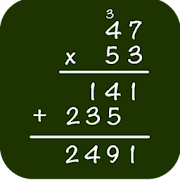# Math: Long Multiplication - Lumos Educational App Store4.61
Price -Free
\$0

#### DESCRIPTION:

The application will teach you how to do a long multiplication. The application is smart: it tries to understand your mistakes and give you appropriate indications. The long multiplication is a fundamental concept of math. Long multiplication is one of the basis of all the skills developed in arithmetics and in math in general. And it makes you learn and consolidate multiplication tables. The application proposes two modes: training and learning. In learning mode, the application shows you how to do the long multiplication step by step. Each step of the math exercise is explained and

#### OVERVIEW:

Math: Long Multiplication is a free educational mobile app By Epsilon Development.It helps students in grades 3rd practice the following standards 3.OA.B.5.

This page not only allows students and teachers download Math: Long Multiplication but also find engaging Sample Questions, Videos, Pins, Worksheets, Books related to the following topics.

1. 3.OA.B.5 : Apply properties of operations as strategies to multiply and divide. Examples: If 6 × 4 = 24 is known, then 4 × 6 = 24 is also known. (Commutative property of multiplication.) 3 × 5 × 2 can be found by 3 × 5 = 15 then 15 × 2 = 30, or by 5 × 2 = 10 then 3 × 10 = 30. (Associative property of multiplication.) Knowing that 8 × 5 = 40 and 8 × 2 = 16, one can find 8 × 7 as 8 × (5 + 2) = (8 × 5) + (8 × 2) = 40 + 16 = 56. (Distributive property.) (Students need not use formal terms for these properties.).

3rd

#### STANDARDS:

3.OA.B.5

Developer: Epsilon Development

Software Version: 16

Category: Education

### RELATED APPSEdSearch WebSearch